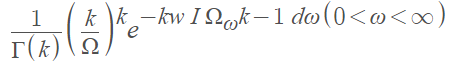# Eulerian Distribution

The term “Eulerian distribution” may refer to:

1. A distribution used in permutation statistics to count descents, or
2. A Type III (p/q, p) distribution.

## Eulerian Distribution in Permutation Statistics

In permutation statistics, the Eulerian distribution is the classical name for the distribution of the descent statistics. A descent in a permutation α1, α2, …, αn is an index I for which αi > αi+1 . The Eulerian number counts the number of permutations in a descent set with exactly k descents .

## Type III (p/q, p) Eulerian Distribution

The term Eulerian distribution may also refer to a Type III (p/q, p) distribution . Kendall’s 1948 Biometrika article, titled “On some modes of population growth leading to R.A. Fisher’s logarithmic series distribution,” concerns relative numbers of animals from different species obtained when random sampling from a heterogeneous population. Fisher assumed that for a certain species, the number of individuals caught in a specific time would be distributed as a Poisson variable with expectation ωt, where ω is the “intrinsic abundance”. The following formula, describing the distribution of ω, is referenced as a Eulerian (or χ2) form:Where

• Ω is the mean value of ω.
• k is a constant parameter.

## References

 Mansour, T. & Munagi, A. (2010). Enumeration of partitions by rises, levels, and descent. In Permutation Patterns. Cambridge University Press.
 Hibi & Tsuchiya. (2019). Algebraic And Geometric Combinatorics On Lattice Polytopes – Proceedings Of The Summer Workshop On Lattice Polytopes. World Scientific.
 Kendall, D. G. (1948). On Some Modes of Population Growth Leading to R. A. Fisher’s Logarithmic Series Distribution. Biometrika, 35(1/2), 6–15. https://doi.org/10.2307/2332624

CITE THIS AS:
Stephanie Glen. "Eulerian Distribution" From StatisticsHowTo.com: Elementary Statistics for the rest of us! https://www.statisticshowto.com/eulerian-distribution/
---------------------------------------------------------------------------Need help with a homework or test question? With Chegg Study, you can get step-by-step solutions to your questions from an expert in the field. Your first 30 minutes with a Chegg tutor is free!